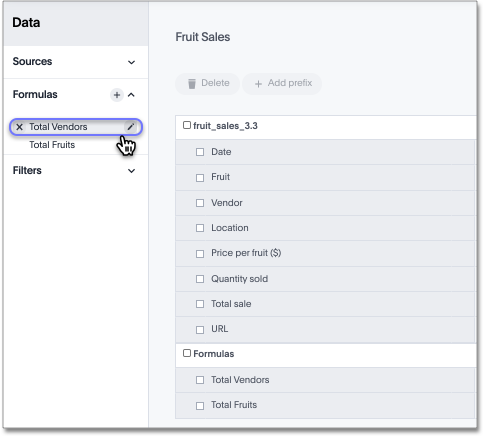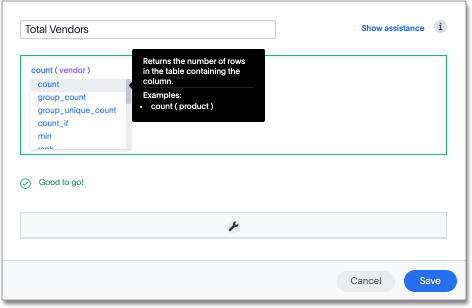Cannot open a saved answer that contains a formula

When working with formulas, keep in mind the data types they return. You may occasionally see unexpected results, or even be unable to open a saved answer, due to problems with data types and formulas.

In this scenario, "data type" refers the data type as defined in the column definition when creating the schema (INT, TIMESTAMP, VARCHAR, and so on).

When you define a formula, the data type it returns is set automatically. This can lead to problems, if you build another formula that uses the output of the first formula as input. This can be hard to understand, so an example will be helpful.

Suppose you have created a worksheet that contains a formula called "weekday" defined as:

day_of_week(date)

The output of that formula is the day of the week (Monday, Tuesday, and so on) returned as a text string (VARCHAR, ATTRIBUTE).

Then suppose you create an answer using the worksheet as a source. And in the answer, you create another formula on top of the formula column in the worksheet. This formula is supposed to return the day of the week that is two days after the given day of the week:

weekday + 2

In this case, you have effectively created a formula on top of another formula. This works fine, so long as the data types in the worksheet formula can work in the answer formula. If not, you may not be able to save the answer, or open it after it has been saved. Here, the second formula you created does not work, because it is invalid. It is trying to add a number to a text string.

If you encounter this issue, you must open the worksheet and edit its formula so that it returns the type expected by the formula that was built on top of it. In this case, a numeric data type.

You must change the underlying worksheet column to use day_number_of_week instead of day_of_week. This is because day_number_of_week returns a numeric data type.

Here are the steps to resolve an issue like this:

1. Open the underlying worksheet that contains the formula whose output data type you need to change.

2. Click the formula name to edit the formula.3. In the Formula Builder, modify the formula, so that it returns the expected data type.

There are data type conversion formulas available to make this easier. To view them and their syntax, open the Formula Assistant, and expand the section called Conversion.4. Make your changes, and saving the formula by clicking Save.

5. Save the worksheet by clicking Save.

6. Now you will be able to open the answer that was created on top of the worksheet.